Skip to content
Related Articles
Lasso Regression in R Programming
• Last Updated : 28 Jul, 2020

Lasso regression is a classification algorithm that uses shrinkage in simple and sparse models(i.e model with fewer parameters). In Shrinkage, data values are shrunk towards a central point like the mean. Lasso regression is a regularized regression algorithm that performs L1 regularization which adds penalty equal to the absolute value of the magnitude of coefficients.

“LASSO” stands for Least Absolute Shrinkage and Selection Operator. Lasso regression is good for models showing high levels of multicollinearity or when you want to automate certain parts of model selection i.e variable selection or parameter elimination. Lasso regression solutions are quadratic programming problems that can best solve with software like RStudio, Matlab, etc. It has the ability to select predictors.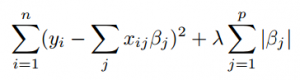The algorithm minimizes the sum of squares with constraint. Some Beta are shrunk to zero that results in a regression model. A tuning parameter lambda controls the strength of the L1 regularization penalty. lambda is basically the amount of shrinkage:

• When lambda = 0, no parameters are eliminated.
• As lambda increases, more and more coefficients are set to zero and eliminated & bias increases.
• When lambda = infinity, all coefficients are eliminated.
• As lambda decreases, variance increases.

Also, If an intercept is included in the model, it is left unchanged. Now let’s implementing Lasso regression in R programming.

### Implementation in R

##### The Dataset

Big Mart dataset consists of 1559 products across 10 stores in different cities. Certain attributes of each product and store have been defined. It consists of 12 features i.e Item_Identifier( is a unique product ID assigned to every distinct item), Item_Weight(includes the weight of the product), Item_Fat_Content(describes whether the product is low fat or not), Item_Visibility(mentions the percentage of the total display area of all products in a store allocated to the particular product), Item_Type(describes the food category to which the item belongs), Item_MRP(Maximum Retail Price (list price) of the product), Outlet_Identifier(unique store ID assigned. It consists of an alphanumeric string of length 6), Outlet_Establishment_Year(mentions the year in which store was established), Outlet_Size(tells the size of the store in terms of ground area covered), Outlet_Location_Type(tells about the size of the city in which the store is located), Outlet_Type(tells whether the outlet is just a grocery store or some sort of supermarket) and Item_Outlet_Sales( sales of the product in the particular store).

 `# Loading data``train = ``fread``(``"Train_UWu5bXk.csv"``)``test = ``fread``(``"Test_u94Q5KV.csv"``)``   ` `# Structure ``str``(train)`

Output: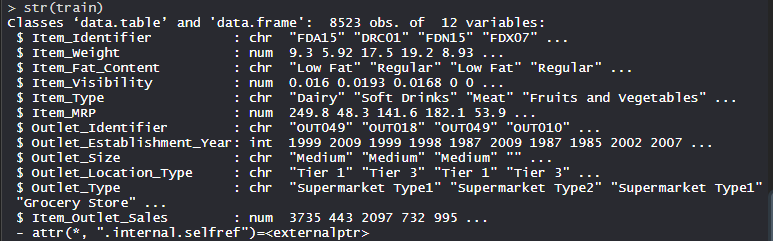##### Performing Lasso Regression on Dataset

Using the Lasso regression algorithm on the dataset which includes 12 features with 1559 products across 10 stores in different cities.

 `# Installing Packages``install.packages``(``"data.table"``)``install.packages``(``"dplyr"``)``install.packages``(``"glmnet"``)``install.packages``(``"ggplot2"``)``install.packages``(``"caret"``)``install.packages``(``"xgboost"``)``install.packages``(``"e1071"``)``install.packages``(``"cowplot"``)`` ` `# load packages``library``(data.table) ``# used for reading and manipulation of data``library``(dplyr)      ``# used for data manipulation and joining``library``(glmnet)     ``# used for regression``library``(ggplot2)    ``# used for ploting ``library``(caret)      ``# used for modeling``library``(xgboost)    ``# used for building XGBoost model``library``(e1071)      ``# used for skewness``library``(cowplot)    ``# used for combining multiple plots `` ` `# Loding datasets``train = ``fread``(``"Train_UWu5bXk.csv"``)``test = ``fread``(``"Test_u94Q5KV.csv"``)`` ` `# Setting test dataset``# Combining datasets``# add Item_Outlet_Sales to test data``test[, Item_Outlet_Sales := ``NA``] `` ` `combi = ``rbind``(train, test)`` ` `# Missing Value Treatment``missing_index = ``which``(``is.na``(combi\$Item_Weight))``for``(i ``in` `missing_index)``{``  ``item = combi\$Item_Identifier[i]``  ``combi\$Item_Weight[i] = ``      ``mean``(combi\$Item_Weight[combi\$Item_Identifier == item], ``                                                 ``na.rm = T)``}`` ` `# Replacing 0 in Item_Visibility with mean``zero_index = ``which``(combi\$Item_Visibility == 0)``for``(i ``in` `zero_index)``{``  ``item = combi\$Item_Identifier[i]``  ``combi\$Item_Visibility[i] = ``      ``mean``(combi\$Item_Visibility[combi\$Item_Identifier == item], ``                                                     ``na.rm = T)``}`` ` `# Label Encoding``# To convert categorical in numerical``combi[, Outlet_Size_num := ``ifelse``(Outlet_Size == ``"Small"``, 0,``                                 ``ifelse``(Outlet_Size == ``"Medium"``,``                                                        ``1, 2))]`` ` `combi[, Outlet_Location_Type_num := ``         ``ifelse``(Outlet_Location_Type == ``"Tier 3"``, 0,``                ``ifelse``(Outlet_Location_Type == ``"Tier 2"``, 1, 2))]`` ` `combi[, ``c``(``"Outlet_Size"``, ``"Outlet_Location_Type"``) := ``NULL``]`` ` `# One Hot Encoding``# To convert categorical in numerical``ohe_1 = ``dummyVars``(``"~."``, data = combi[, -``c``(``"Item_Identifier"``, ``                                          ``"Outlet_Establishment_Year"``,``                                          ``"Item_Type"``)], fullRank = T)``ohe_df = ``data.table``(``predict``(ohe_1, combi[, -``c``(``"Item_Identifier"``, ``                                           ``"Outlet_Establishment_Year"``,``                                           ``"Item_Type"``)]))`` ` `combi = ``cbind``(combi[, ``"Item_Identifier"``], ohe_df)`` ` `# Remove skewness``skewness``(combi\$Item_Visibility) ``skewness``(combi\$price_per_unit_wt)`` ` `# log + 1 to avoid division by zero``combi[, Item_Visibility := ``log``(Item_Visibility + 1)] `` ` `# Scaling and Centering data``num_vars = ``which``(``sapply``(combi, is.numeric)) ``# index of numeric features``num_vars_names = ``names``(num_vars)`` ` `combi_numeric = combi[, ``setdiff``(num_vars_names, ``                              ``"Item_Outlet_Sales"``),``                               ``with = F]`` ` `prep_num = ``preProcess``(combi_numeric, ``                      ``method=``c``(``"center"``, ``"scale"``))``combi_numeric_norm = ``predict``(prep_num, combi_numeric)`` ` `# removing numeric independent variables``combi[, ``setdiff``(num_vars_names, ``                ``"Item_Outlet_Sales"``) := ``NULL``]``combi = ``cbind``(combi, combi_numeric_norm)`` ` `# splitting data back to train and test``train = combi[1:``nrow``(train)]``test = combi[(``nrow``(train) + 1):``nrow``(combi)]`` ` `# Removing Item_Outlet_Sales``test[, Item_Outlet_Sales := ``NULL``] `` ` `# Model Building :Lasso Regression``set.seed``(123)``control = ``trainControl``(method =``"cv"``, number = 5)``Grid_la_reg = ``expand.grid``(alpha = 1,``              ``lambda = ``seq``(0.001, 0.1, by = 0.0002))`` ` `# Training lasso regression model``lasso_model = ``train``(x = train[, -``c``(``"Item_Identifier"``, ``                               ``"Item_Outlet_Sales"``)],``                    ``y = train\$Item_Outlet_Sales,``                    ``method = ``"glmnet"``,``                    ``trControl = control,``                    ``tuneGrid = Grid_reg``                    ``)``lasso_model`` ` `# mean validation score``mean``(lasso_model\$resample\$RMSE)`` ` `# Plot``plot``(lasso_model, main = ``"Lasso Regression"``)`

Output:

• Model lasso_model: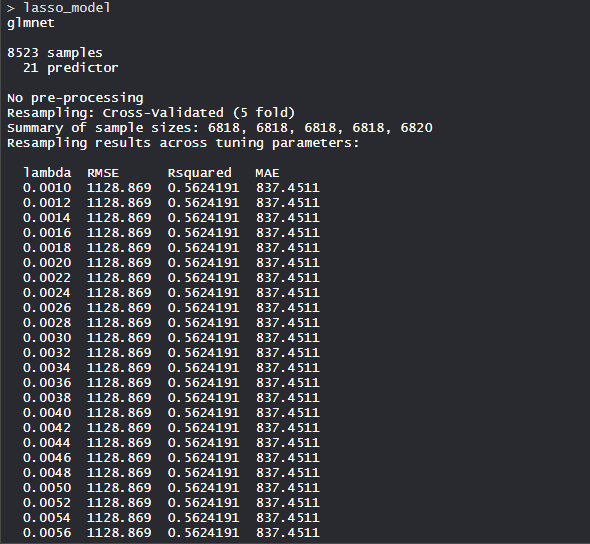The Lasso regression model uses the alpha value as 1 and lambda value as 0.1. RMSE was used to select the optimal model using the smallest value.

• Mean validation score: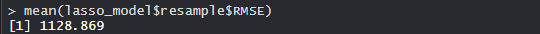The mean validation score of the model is 1128.869.

• Plot: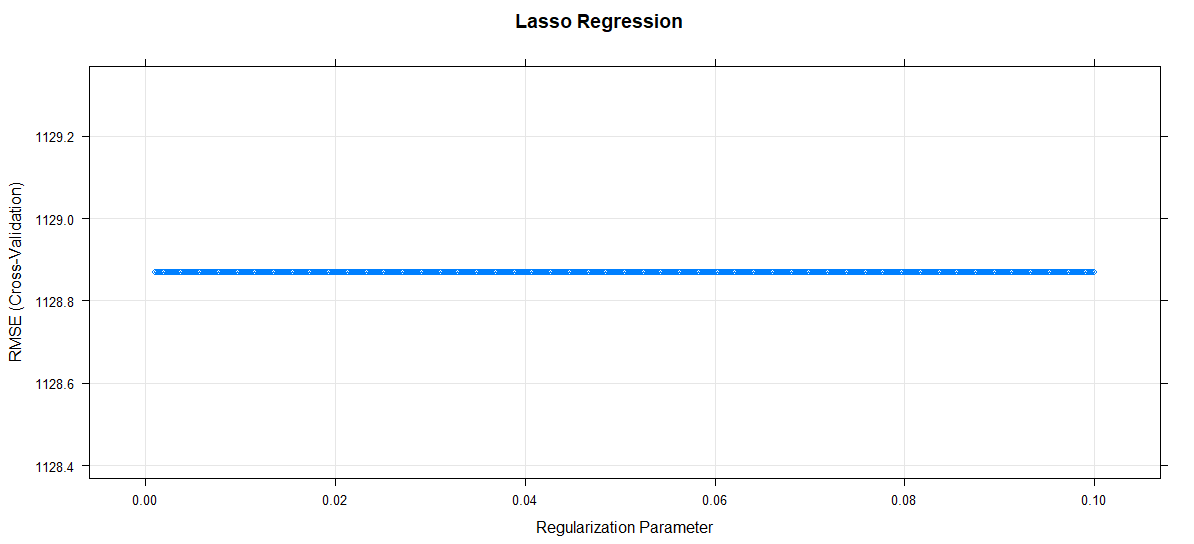The regularization parameter increases, RMSE remains constant.

So, Lasso regression finds its applications in many sectors of industry and used with full capacity.

My Personal Notes arrow_drop_up